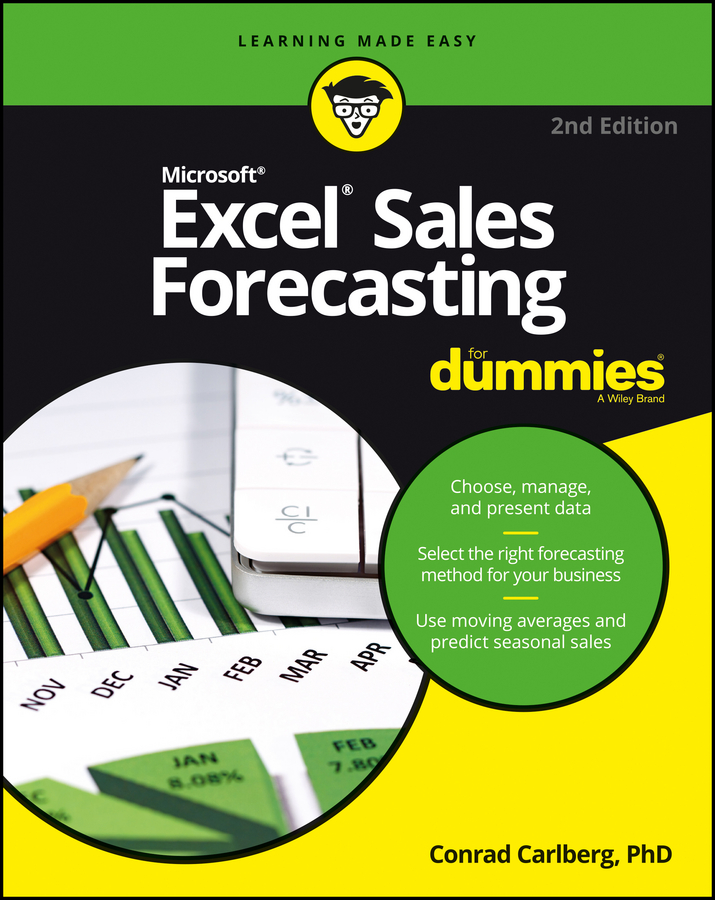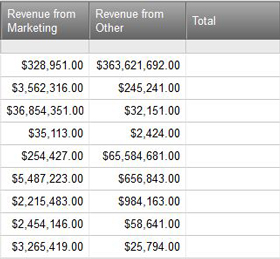##### Excel Sales Forecasting For DummiesJust like Microsoft Excel or other spreadsheet programs, Smartsheet enables you to use formulas to automatically calculate data within a sheet. You can perform basic operations, such as addition or multiplication, or more advanced operations, such as calculating averages.

Smartsheet’s formula syntax (that is, how formulas are written) differs a bit from Excel, but the basics are the same.

As an example, say you have two different columns that you need to add together across the rows (see the example in Figure 1). Follow these steps to create an equation to add the two columns together:

1. Click the first cell in the Total column.

2. Type an equal sign.

3. Click the first cell under Revenue from Marketing. You see the column name and cell number appear after the equal sign.

4. Type a plus sign.

5. Hold down the Shift key and click the first cell under the Revenue from Other column. You see the column name and cell number appear after the plus sign.

6. Hit the Enter key. The sum of the two columns appears in first cell of the Total column.

7. To use this formula for the rest of the rows, click the first cell in the Total column.

8. Hover your mouse over the bottom-right corner of the cell. You see a big plus sign. Click and drag the corner down the rest of the cells of the column.

Your formula populates in the other Total cells, and the cells you’re adding together automatically update based on which row the formula appears in.Figure 1: Add these columns together.

Source: smartsheet.com

You don’t necessarily have to type in the formula yourself, if Smartsheet already has a built-in function for it. In this example, you can use the SUM function instead.

1. Click the first cell of the Total column.

2. Click the f(x) button on the left and choose SUM from the drop-down menu.

3. Click the first cell in the Revenue from Marketing column, and then hold down the Shift key and click the first cell in the Revenue from Other column.

4. Hit Enter. You see the total appear. Use the same method above to drag-fill the formula into the rest of the Total cells.

Smartsheet supports many different types of formulas. You can view examples of all these formulas here.